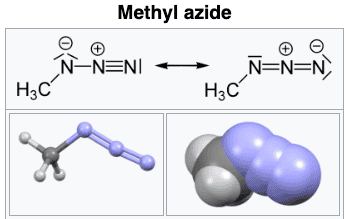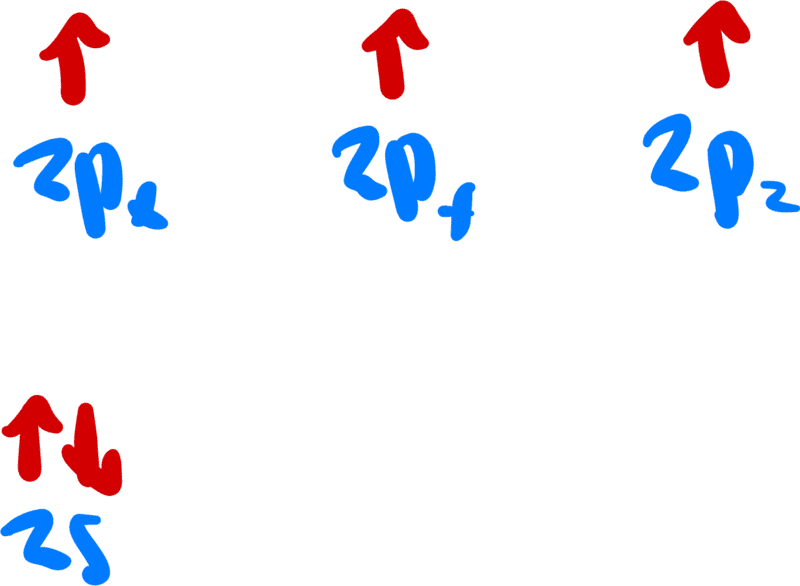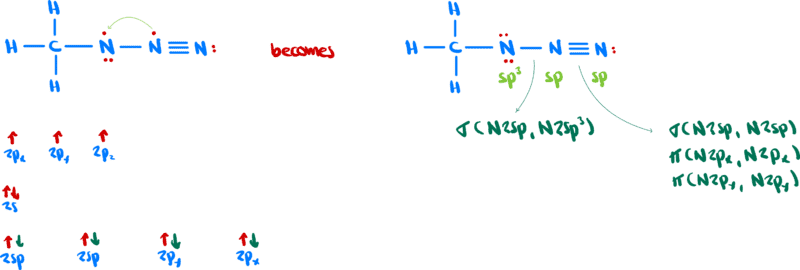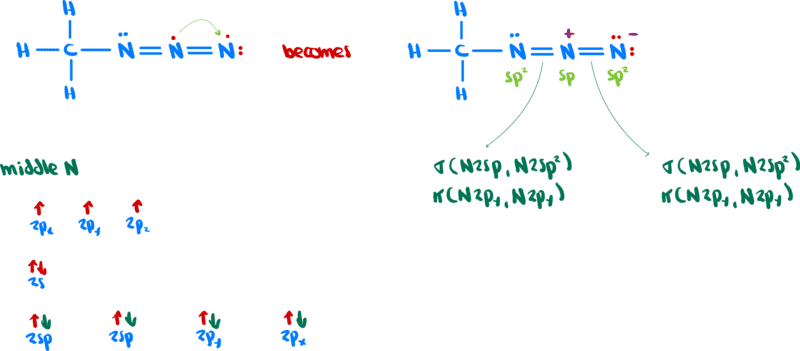# How to understand hybridization in ##CH_3NNN##?

• Chemistry
• zenterix

#### zenterix

Homework Statement
I'm solving a problem set from MIT OCW's "Principles of Chemical Science". The topic is valence bond theory.

There is a problem that asks us to identify the hybridization of the middle nitrogen atom in the molecule

##CH_3NNN##
Relevant Equations
The process I usually go through is to draw a lewis structure, use VSEPR to find the electron arrangement and the molecular shape, and then based on the number of atoms and lone pairs on an atom I choose a hybridization.
The problem is that I am not sure how the ##NNN## portion of the molecule fits together.

After looking on Wikipedia, it seems the structure isI am not sure about the reasoning that leads one to the above (resonant?) structures. Let's call the leftmost structure A and the rightmost structure B.

My question is what is the hybridization of the middle nitrogen and how do we think about the distribution of electrons among the molecular orbitals.

A nitrogen alone has the valence electron configurationNow, in both the structures, there is are no unpaired electrons or any lone pairs on the middle nitrogen because, for some reason that I am unaware of, one of the electrons on the middle nitrogen goes over to another nitrogen.

In structure A, we haveSimilarly, in structure B we haveThe middle nitrogen has the same hybridization in both structures. The difference is that in one case two ##\pi## bonds are formed with another nitrogen (for a triple bond) and in the other case there is one #\pi# bond with each nitrogen (for two double bonds).

My question, first of all, is if this analysis is correct.

Second of all, how do we know when a nitrogen will give up an electron as is happening here?

Now, I have a rudimentary understanding that in some models electrons are delocalized, but I am not aware that that is the case in valence bond theory.

#### Attachments

•dextercioby
My question, first of all, is if this analysis is correct
Yes, looks good to me.
Second of all, how do we know when a nitrogen will give up an electron as is happening here?
Try drawing Lewis structures other than the two resonance structures in your OP. Evaluate the formal charges on each of the atoms. What does this tell you about the contributions of these resonance structures to the overall structure of the molecule?
Now, I have a rudimentary understanding that in some models electrons are delocalized, but I am not aware that that is the case in valence bond theory.
The important thing in VB is that—loosely speaking—the actual molecular structure isn’t necessarily a single Lewis structure; rather, the molecular structure is a weighted combination of each of the resonance structures. The more “reasonable” structures are usually weighted more heavily than the wackier looking structures. In some sense, this is intuitive, but if you find a Lewis structure whose atoms have lots and lots of formal charge, chances are that represents a very high-energy, and therefore only slightly contributing, piece of the overall structure.

•DrClaude and zenterix
The article by Hilberty might be interesting: https://www.sciencedirect.com/science/article/pii/0022286093870222
He tests the importance of different valence bond structures using ab initio valence bond calculations.
Maybe somewhat easier to understand is the article by Klapötke:
https://chemistry-europe.onlinelibr...sKqYSf1LQo8Iw6_-6BNPxA5aMuR6y2_09hFp9rMbQyXpQ

While different hybridization schemes seem to lead to comparable conclusions, the important point brought out in both articles is the importance of "long bond structures" - bonds between non-neighbouring atoms - participating in resonance.

Last edited:
•dextercioby
I pondered a little bit about the Methylazide. I think it is easiest, to consider the hybridization of N(1) (the one which is bound to the methyl group) as sp2, N(2) and N(3) as sp. Then there is a sigma bond a pi bond within the plane between N(2) and N(3) and a lone pair in this plane on N(1). On each of the three nitrogens there is a p-pi orbital perpendicular to the plane. These 3 orbitals hold 4 electrons in total, so it is a 4-electron-3-center bond. The pi system is isoelectronic to the one of the allyl-anion (C3H5-). I tried to calculate the energy of the pi system using classical VB theory. In this approximation, orbitals are assumed to be almost orthogonal, but some non-orthogonality is needed to explain the bond. E.g. the bonding in a single two-electron bond between orbitals a and b is due to exchange matrix elements ##K_{ab}\sim<a|b> <a| h1+h2| b> ## where h1 and h2 are the one electron hamiltonians of the two atoms. Now in the 3 center problem with orbitals p-orbitals a, b, and c one structure may be derived from a(1)a(2)b(3)c(4) by combining with spin functions and antisymmetrizing where the (i) are the indices of the electrons. With a singlet pairing between a singlet pairing between b(3) c(4) a bond arises with is somewhat weakened by the interaction with the lone pair a(1)a(2). If resonance is taken into account something interesting happens if the Dewar structure derived from a(1)b(2)b(3)c(4) is taken into account. Namely considering ##<aabc|H|abbc> \sim <a|h1+h2|b>##, i.e. the resonance matrix element is stronger than a normal exchange matrix element as it lacks an overlapp integral ##<a|b>## as compared to ##K_{ab}##.

The old article by Simonetta, M., Heilbronner, E. Valence bond treatment of systems involving orbital degeneracy. Theoret. Chim. Acta 2, 228–246 (1964). https://doi.org/10.1007/BF00528282
is probably as far as one can get using oldfashioned semiempirical valence bond theory. The result for the allyl anion should be comparable to the pi system in methylazide. The total strength of the 4-electron-3-center bond is about 1.56 times the strength of a single bond. In this article they assume that the matrix element ##\gamma:=<aabc| H | abbc>\approx K_{ab}=:\alpha##, where alpha and gamma are the names given to these quantities in the article.

•dextercioby